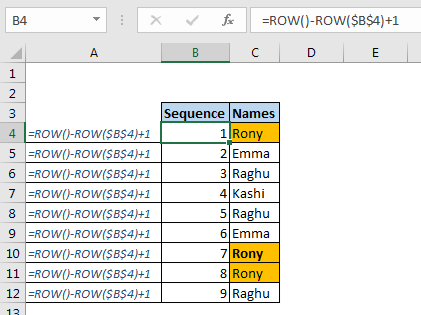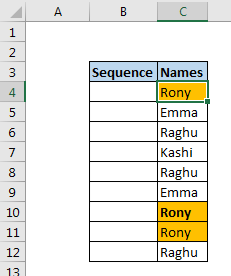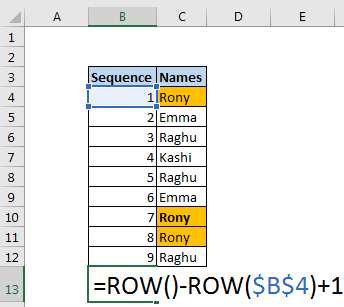# How To Get Sequential Row Number in Excel

Sometimes we need to get a sequential row number of in table, it can be for serial number or anything else. In this article, we will learn how to number rows in excel from the start of data.Generic Formula

=ROW()-ROW(absolute reference of start cell)+1

Absolute reference of start cell: it is the absolute reference of cell in which you are writing this formula first. For example, if the serial number starts from cell A2 then it is \$A\$2.
Let’s see an example to make things clear.

Example: Get sequential numbering using excel formula
Here I have some names in range C4:C12. In B4:B12, I want to have a sequence or serial number. So the serial number will start with B4.Apply the above generic formula here to get sequence numbers in excel.

=ROW-ROW(\$B\$4)+1

Drag it down to get a sequence of numbers, starting from 1 and increasing from 1 with each row.How does it work?
It is easy.
The ROW function returns the row number of cells it is written in if no variable is passed. Eg. 2 if we have ROW() written in F2. If we supply a cell reference to ROW function it returns the row number of a given cell reference.
Here, we have ROW()-ROW(\$B\$4)+1 in cell B4. this solves to 4-4+1. Which gives 1. When we copy this formula below in cell B5, the formula will be unchanged but it will solve to 5-4+1. This will give 2. This will happen to all cells you will copy this formula in. and this is how we get serial number automatically.
So yeah guys, this is how you can have dynamic row numbering in excel. Any changes below or above rows will not affect the formula and will continue to have your sequential numbers without changing anything in the formula. If you have any doubts regarding this article or any other excel topic, let me know in the comments section below.

Related Articles:

How To Count Unique Text in Excel

Popular Articles:

The VLOOKUP Function in Excel

COUNTIF in Excel 2016

How to Use SUMIF Function in Excel

Terms and Conditions of use

The applications/code on this site are distributed as is and without warranties or liability. In no event shall the owner of the copyrights, or the authors of the applications/code be liable for any loss of profit, any problems or any damage resulting from the use or evaluation of the applications/code.